Скачать презентацию AC Circuits AC Sources Often electrical signals

9d5657fc467d849418f4c704bd8cbc3a.ppt

• Количество слайдов: 26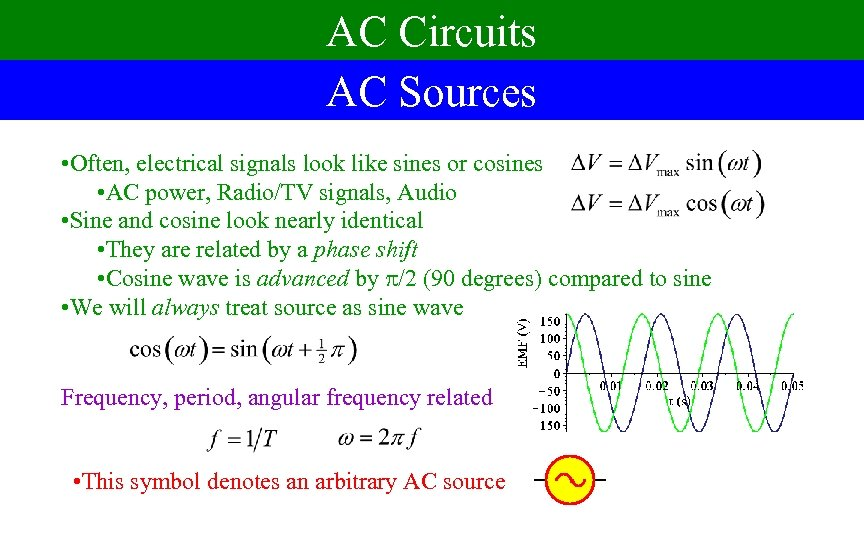AC Circuits AC Sources • Often, electrical signals look like sines or cosines • AC power, Radio/TV signals, Audio • Sine and cosine look nearly identical • They are related by a phase shift • Cosine wave is advanced by /2 (90 degrees) compared to sine • We will always treat source as sine wave Frequency, period, angular frequency related • This symbol denotes an arbitrary AC source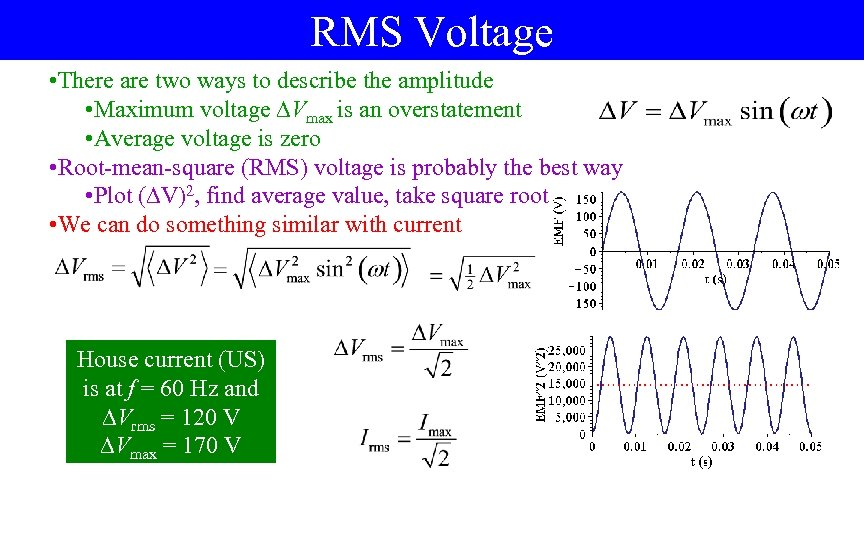RMS Voltage • There are two ways to describe the amplitude • Maximum voltage Vmax is an overstatement • Average voltage is zero • Root-mean-square (RMS) voltage is probably the best way • Plot ( V)2, find average value, take square root • We can do something similar with current House current (US) is at f = 60 Hz and Vrms = 120 V Vmax = 170 V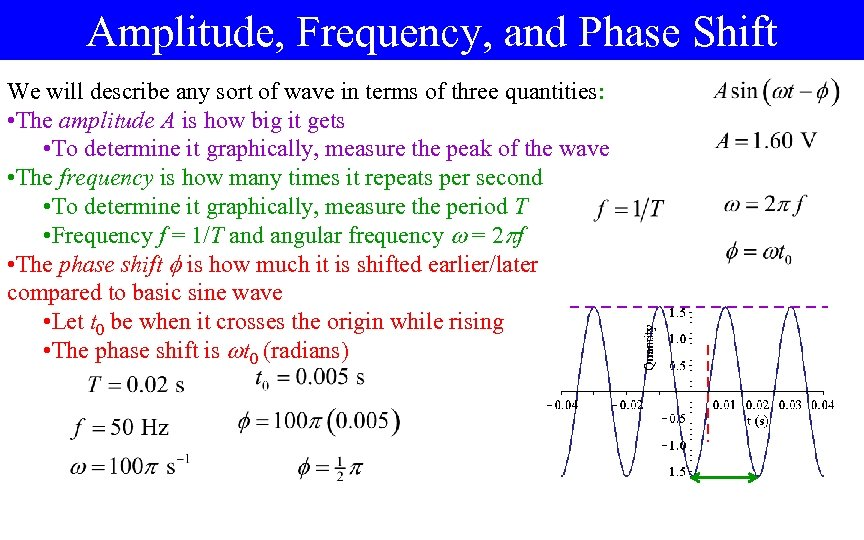Amplitude, Frequency, and Phase Shift We will describe any sort of wave in terms of three quantities: • The amplitude A is how big it gets • To determine it graphically, measure the peak of the wave • The frequency is how many times it repeats per second • To determine it graphically, measure the period T • Frequency f = 1/T and angular frequency = 2 f • The phase shift is how much it is shifted earlier/later compared to basic sine wave • Let t 0 be when it crosses the origin while rising • The phase shift is t 0 (radians)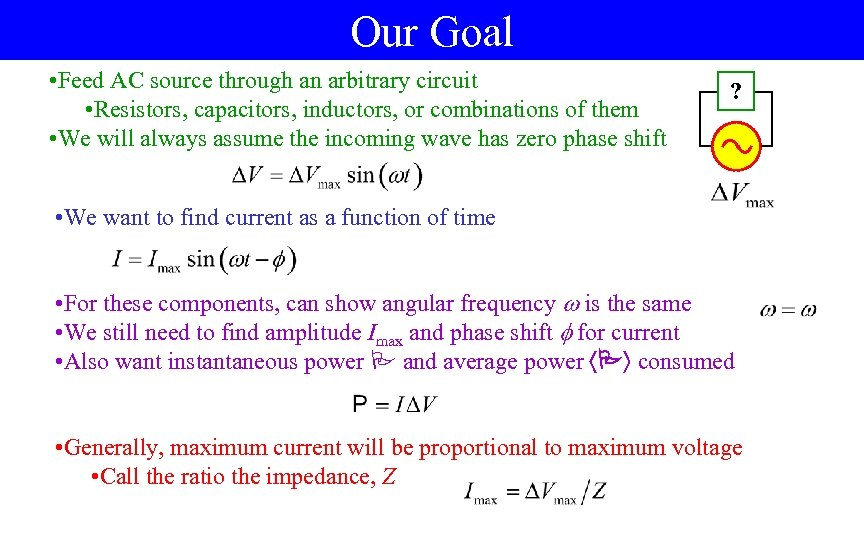Our Goal • Feed AC source through an arbitrary circuit • Resistors, capacitors, inductors, or combinations of them • We will always assume the incoming wave has zero phase shift ? • We want to find current as a function of time • For these components, can show angular frequency is the same • We still need to find amplitude Imax and phase shift for current • Also want instantaneous power P and average power P consumed • Generally, maximum current will be proportional to maximum voltage • Call the ratio the impedance, Z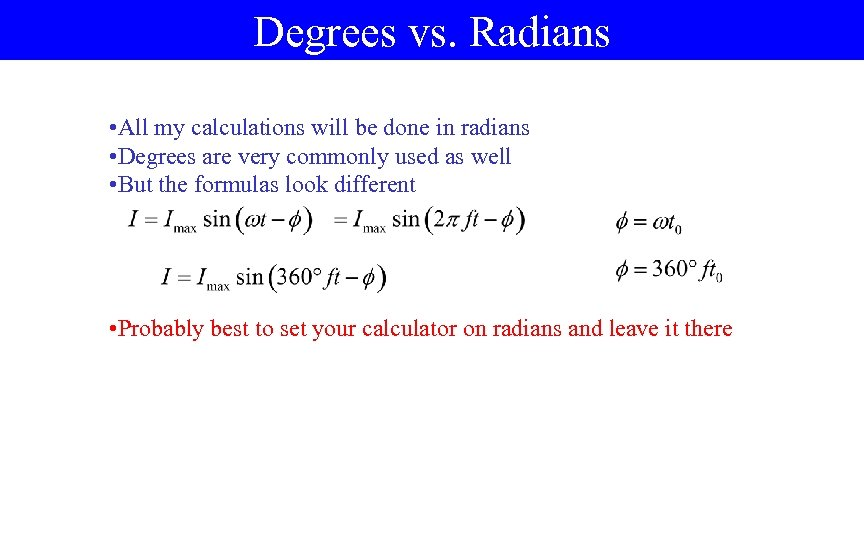Degrees vs. Radians • All my calculations will be done in radians • Degrees are very commonly used as well • But the formulas look different • Probably best to set your calculator on radians and leave it there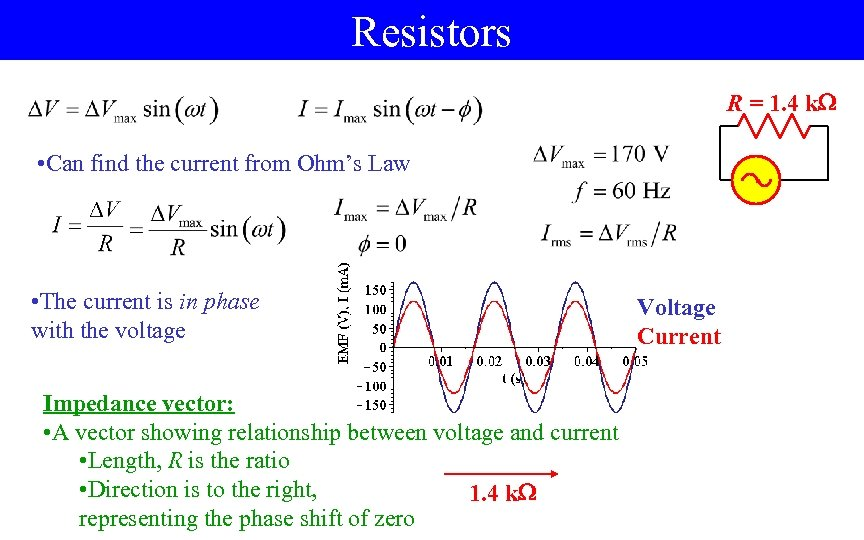Resistors R = 1. 4 k • Can find the current from Ohm’s Law • The current is in phase with the voltage Impedance vector: • A vector showing relationship between voltage and current • Length, R is the ratio • Direction is to the right, 1. 4 k representing the phase shift of zero Voltage Current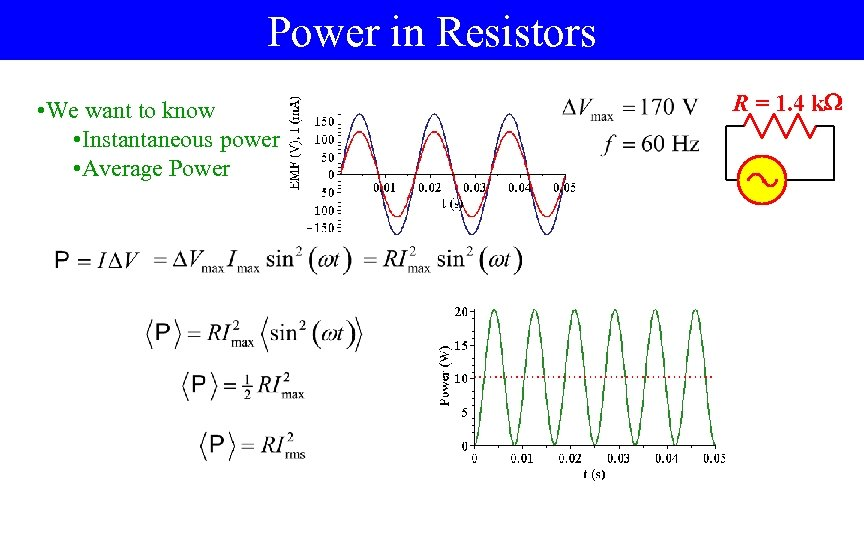Power in Resistors • We want to know • Instantaneous power • Average Power R = 1. 4 k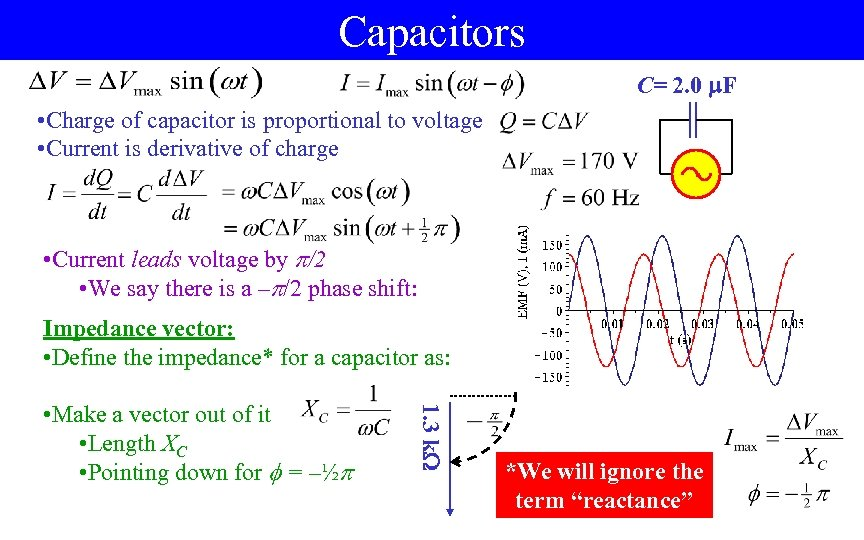Capacitors C= 2. 0 F • Charge of capacitor is proportional to voltage • Current is derivative of charge • Current leads voltage by /2 • We say there is a – /2 phase shift: Impedance vector: • Define the impedance* for a capacitor as: 1. 3 k • Make a vector out of it • Length XC • Pointing down for = –½ *We will ignore the term “reactance”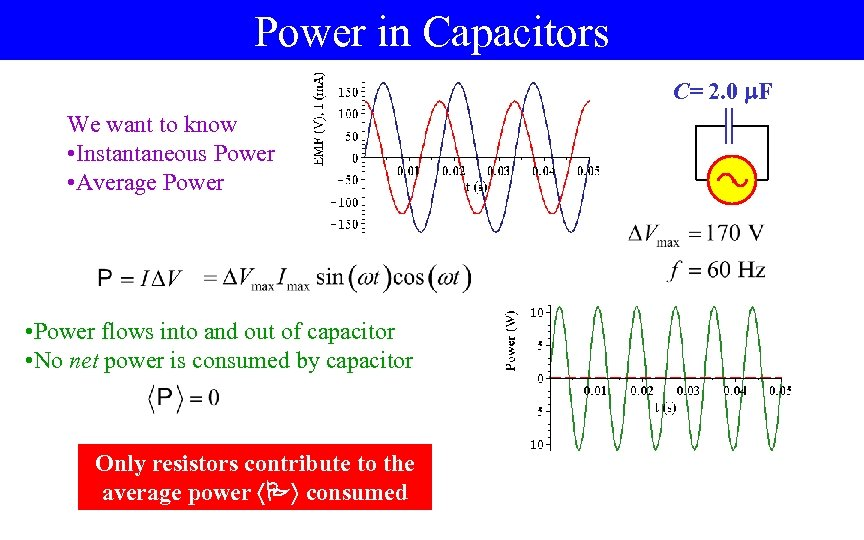Power in Capacitors C= 2. 0 F We want to know • Instantaneous Power • Average Power • Power flows into and out of capacitor • No net power is consumed by capacitor Only resistors contribute to the average power P consumed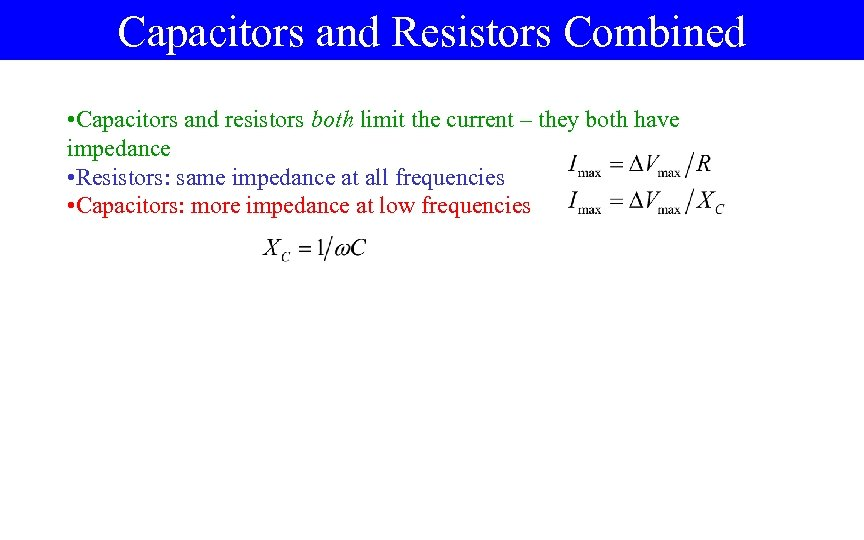Capacitors and Resistors Combined • Capacitors and resistors both limit the current – they both have impedance • Resistors: same impedance at all frequencies • Capacitors: more impedance at low frequencies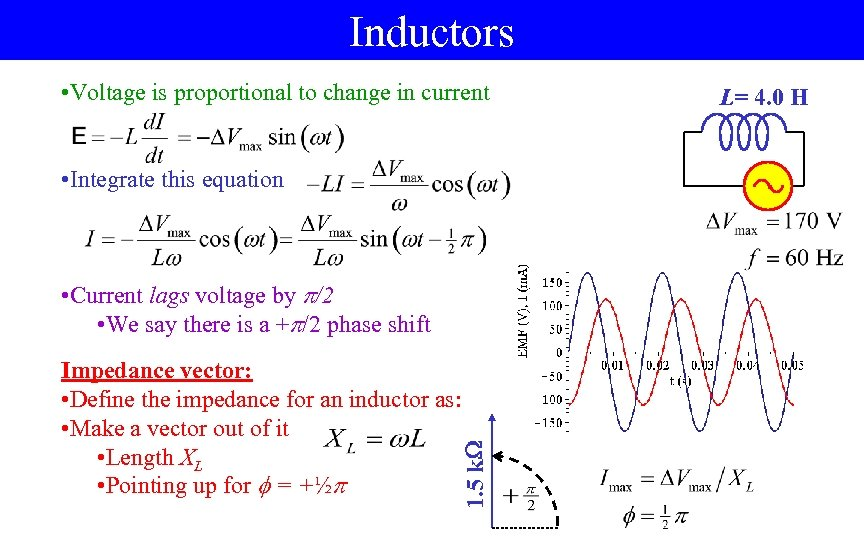Inductors • Voltage is proportional to change in current • Integrate this equation Impedance vector: • Define the impedance for an inductor as: • Make a vector out of it • Length XL • Pointing up for = +½ 1. 5 k • Current lags voltage by /2 • We say there is a + /2 phase shift L= 4. 0 H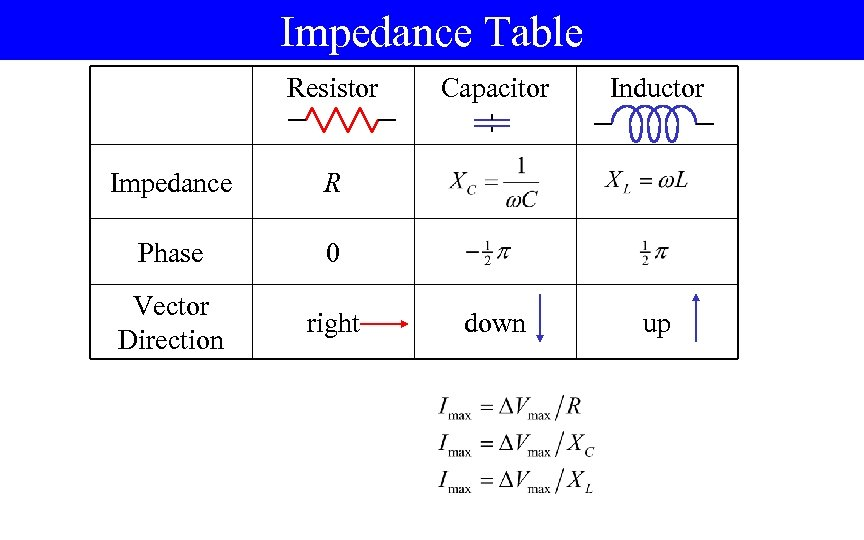Impedance Table Resistor Impedance right down up 0 Vector Direction Inductor R Phase Capacitor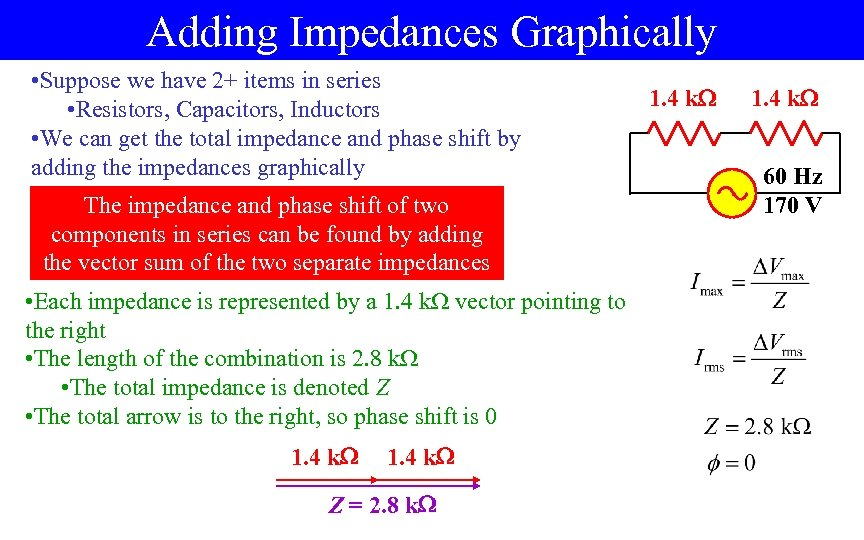Adding Impedances Graphically • Suppose we have 2+ items in series • Resistors, Capacitors, Inductors • We can get the total impedance and phase shift by adding the impedances graphically The impedance and phase shift of two components in series can be found by adding the vector sum of the two separate impedances • Each impedance is represented by a 1. 4 k vector pointing to the right • The length of the combination is 2. 8 k • The total impedance is denoted Z • The total arrow is to the right, so phase shift is 0 1. 4 k Z = 2. 8 k 1. 4 k 60 Hz 170 V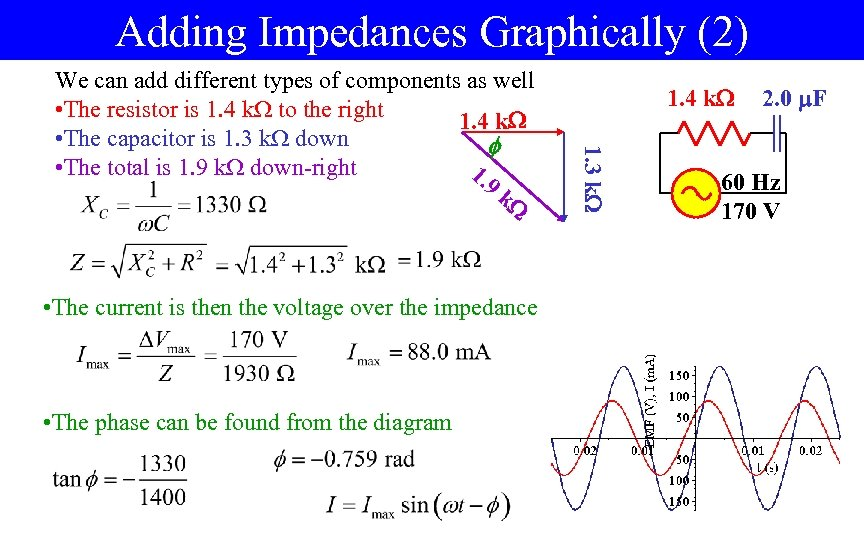Adding Impedances Graphically (2) • The current is then the voltage over the impedance • The phase can be found from the diagram 1. 4 k 1. 3 k We can add different types of components as well • The resistor is 1. 4 k to the right 1. 4 k • The capacitor is 1. 3 k down • The total is 1. 9 k down-right 1. 9 k 2. 0 F 60 Hz 170 V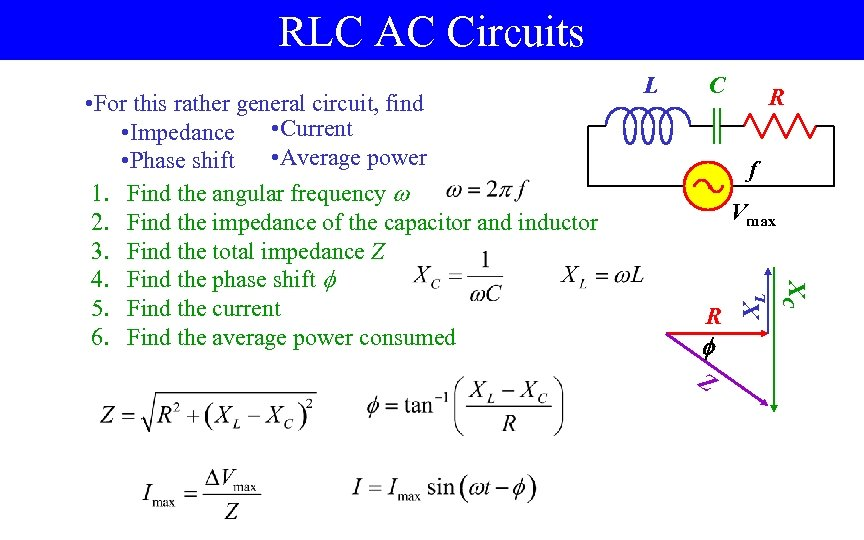RLC AC Circuits C R f R Z XL Vmax XC • For this rather general circuit, find • Impedance • Current • Average power • Phase shift 1. Find the angular frequency 2. Find the impedance of the capacitor and inductor 3. Find the total impedance Z 4. Find the phase shift 5. Find the current 6. Find the average power consumed L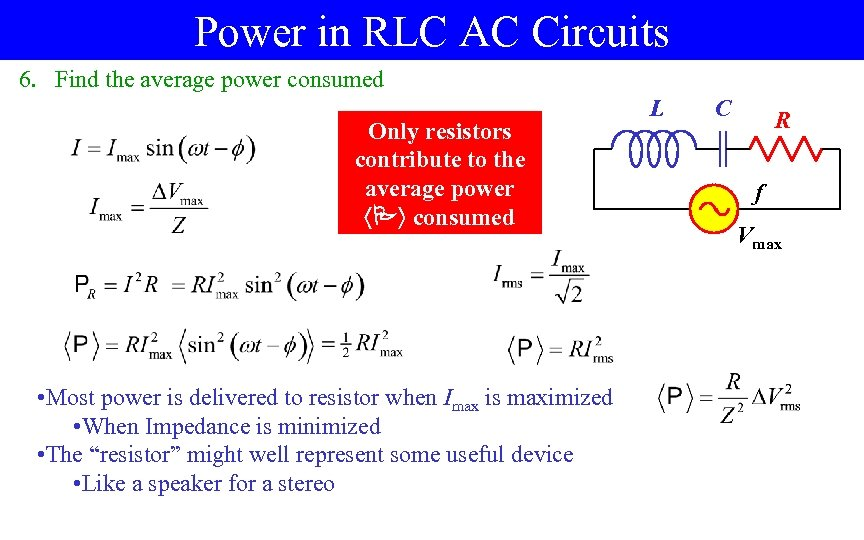Power in RLC AC Circuits 6. Find the average power consumed Only resistors contribute to the average power P consumed • Most power is delivered to resistor when Imax is maximized • When Impedance is minimized • The “resistor” might well represent some useful device • Like a speaker for a stereo L C R f Vmax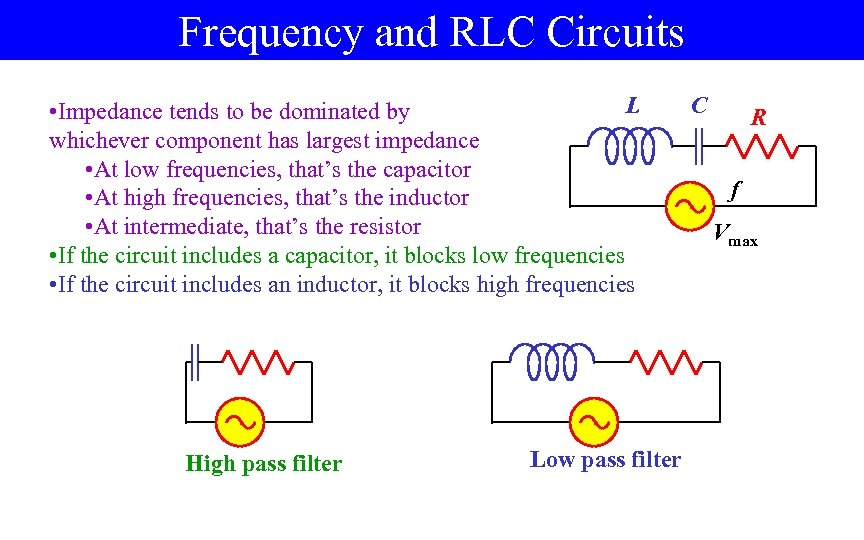Frequency and RLC Circuits L • Impedance tends to be dominated by whichever component has largest impedance • At low frequencies, that’s the capacitor • At high frequencies, that’s the inductor • At intermediate, that’s the resistor • If the circuit includes a capacitor, it blocks low frequencies • If the circuit includes an inductor, it blocks high frequencies High pass filter Low pass filter C R f Vmax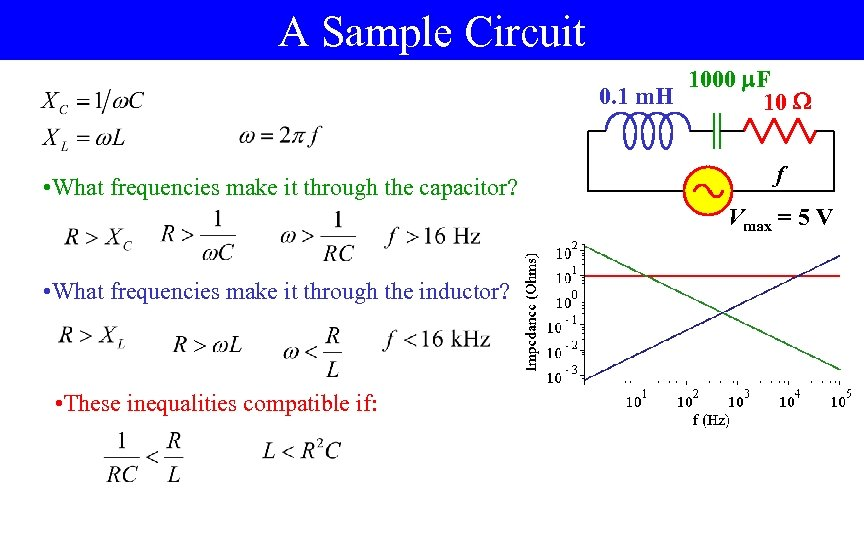A Sample Circuit 1000 F 0. 1 m. H 10 • What frequencies make it through the capacitor? f Vmax = 5 V • What frequencies make it through the inductor? • These inequalities compatible if: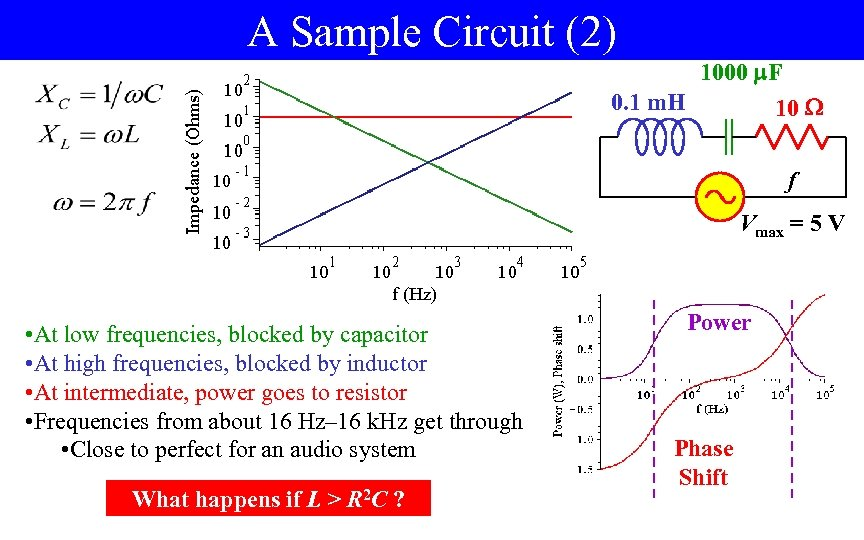A Sample Circuit (2) 1000 F 0. 1 m. H 10 f Vmax = 5 V • At low frequencies, blocked by capacitor • At high frequencies, blocked by inductor • At intermediate, power goes to resistor • Frequencies from about 16 Hz– 16 k. Hz get through • Close to perfect for an audio system What happens if L > R 2 C ? Power Phase Shift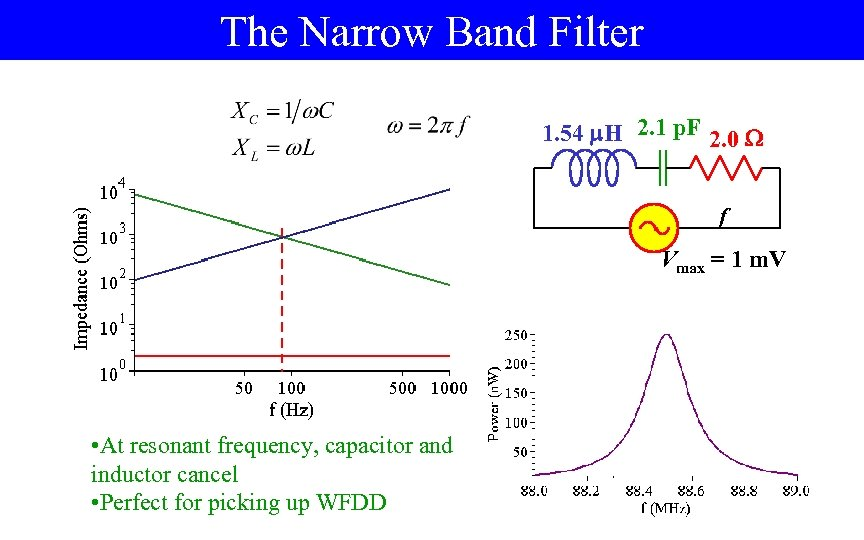The Narrow Band Filter 1. 54 H 2. 1 p. F 2. 0 f Vmax = 1 m. V • At resonant frequency, capacitor and inductor cancel • Perfect for picking up WFDD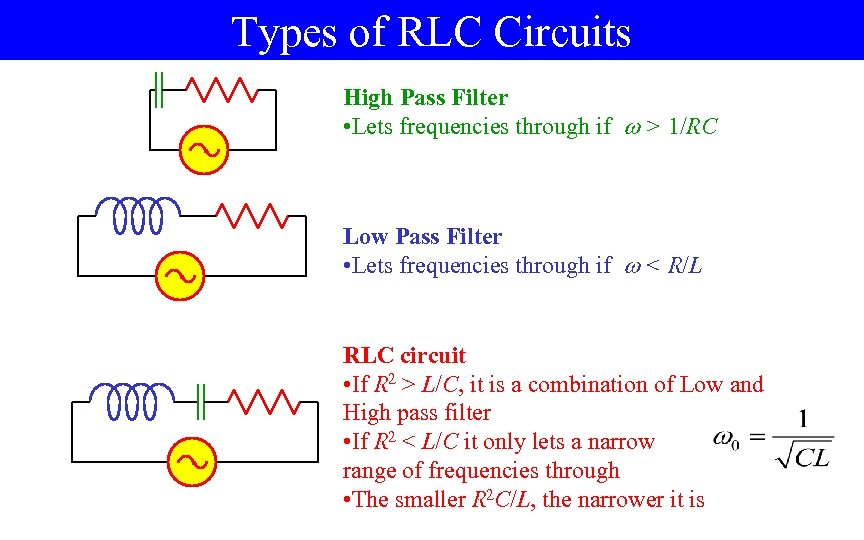Types of RLC Circuits High Pass Filter • Lets frequencies through if > 1/RC Low Pass Filter • Lets frequencies through if < R/L RLC circuit • If R 2 > L/C, it is a combination of Low and High pass filter • If R 2 < L/C it only lets a narrow range of frequencies through • The smaller R 2 C/L, the narrower it is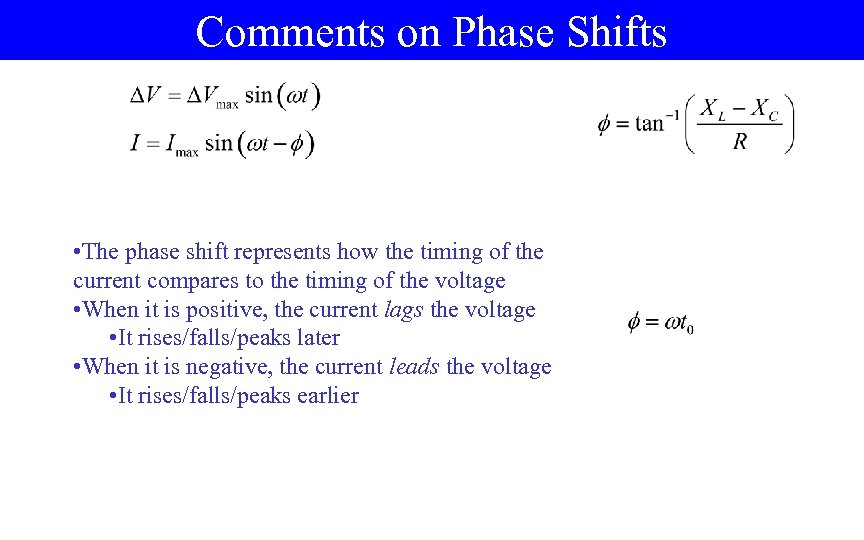Comments on Phase Shifts • The phase shift represents how the timing of the current compares to the timing of the voltage • When it is positive, the current lags the voltage • It rises/falls/peaks later • When it is negative, the current leads the voltage • It rises/falls/peaks earlier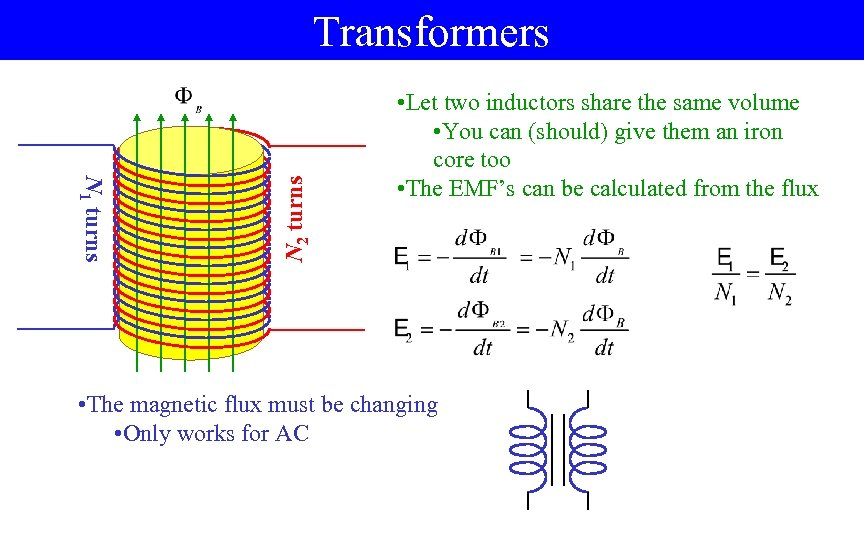N 1 turns N 2 turns Transformers • Let two inductors share the same volume • You can (should) give them an iron core too • The EMF’s can be calculated from the flux • The magnetic flux must be changing • Only works for AC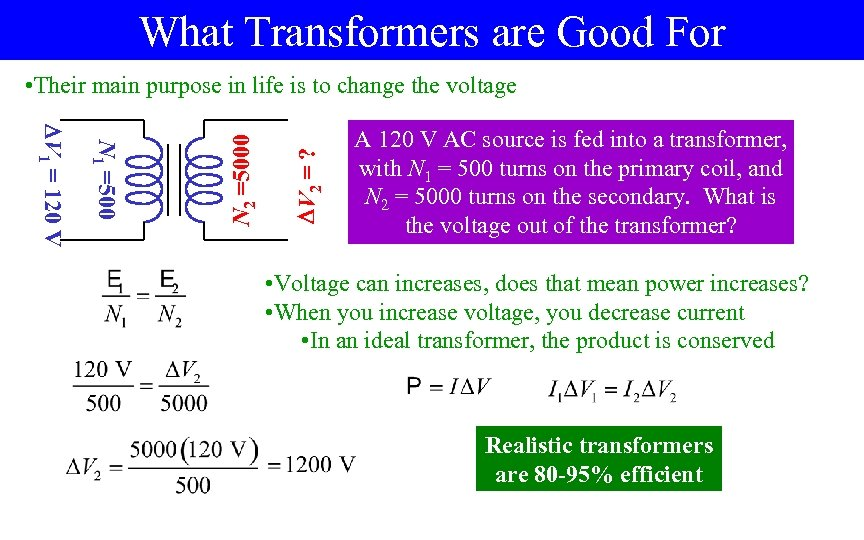What Transformers are Good For V 2 = ? N 1 =500 V 1 = 120 V N 2 =5000 • Their main purpose in life is to change the voltage A 120 V AC source is fed into a transformer, with N 1 = 500 turns on the primary coil, and N 2 = 5000 turns on the secondary. What is the voltage out of the transformer? • Voltage can increases, does that mean power increases? • When you increase voltage, you decrease current • In an ideal transformer, the product is conserved Realistic transformers are 80 -95% efficient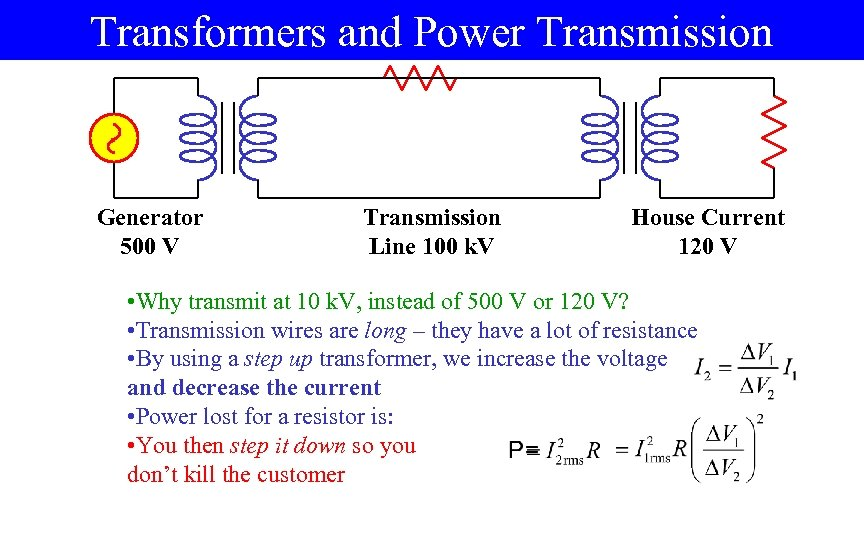Transformers and Power Transmission Generator 500 V Transmission Line 100 k. V House Current 120 V • Why transmit at 10 k. V, instead of 500 V or 120 V? • Transmission wires are long – they have a lot of resistance • By using a step up transformer, we increase the voltage and decrease the current • Power lost for a resistor is: • You then step it down so you don’t kill the customer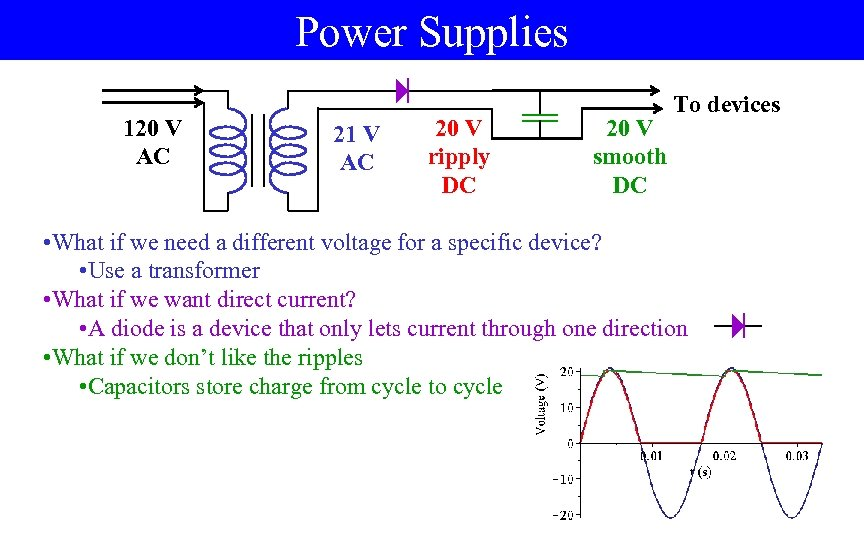Power Supplies 120 V AC 21 V AC 20 V ripply DC 20 V smooth DC To devices • What if we need a different voltage for a specific device? • Use a transformer • What if we want direct current? • A diode is a device that only lets current through one direction • What if we don’t like the ripples • Capacitors store charge from cycle to cycle